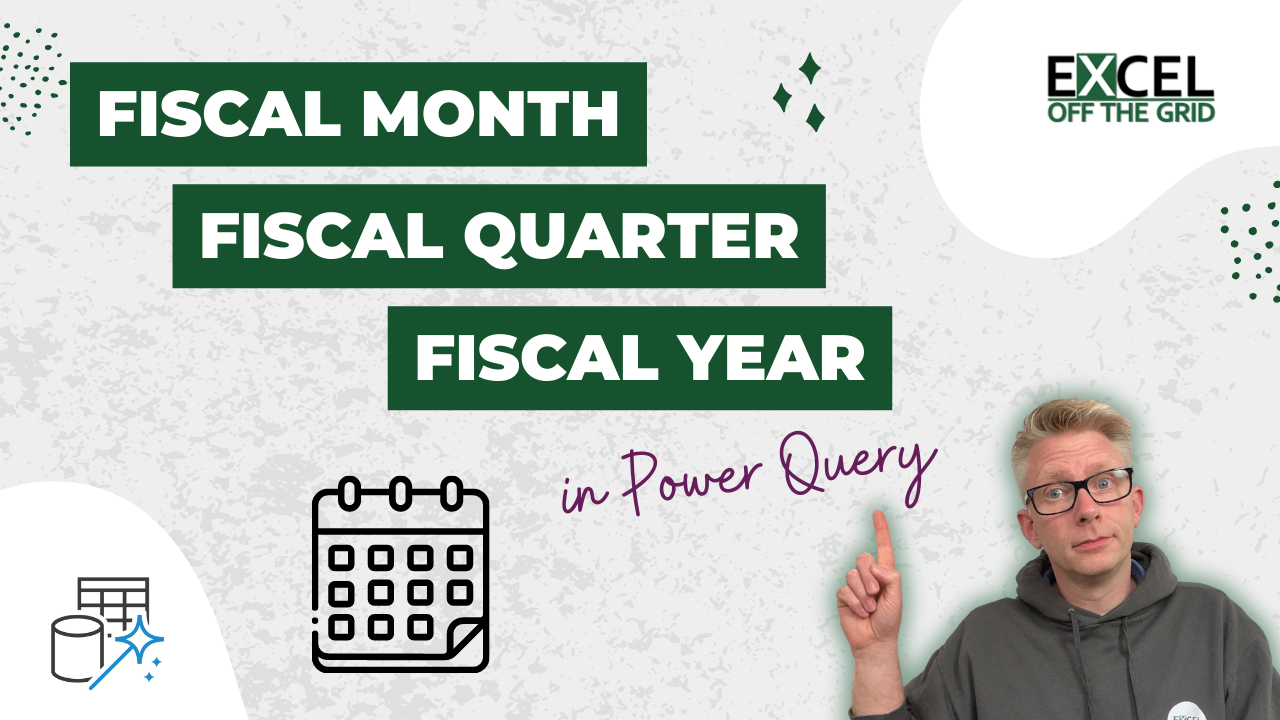Get our FREE VBA eBook of the 30 most useful Excel VBA macros.

Automate Excel so that you can save time and stop doing the jobs a trained monkey could do.

# How to add fiscal Month, Quarter or Year Column in Power QueryOver the last few months, I’ve been asked several times how to add a fiscal month, fiscal quarter, or fiscal year column in Power Query. So, I decided to note down the method; therefore, I don’t have to keep reminding myself how I did it last time. The good news is that it’s reasonably straightforward. Let’s see what we need to do.

Watch the video

Contents

## Data

For this example, I’m starting with a simple data set.

All of these dates are in the dd/mm/yyyy format. Depending on your regional setting, they may be in another date format.

Throughout this post, I’m assuming:

• We are using a standard calendar for month ends. I’ll cover non-standard calendars in a future post.
• The year-end is March (i.e., Period 3)

## Add a fiscal month column

There are several ways to calculate the fiscal month; I’m going to demonstrate the easiest to understand.

In Power Query, click Add Column > Custom Column.

In the Custom Column dialog box, enter the following formula:

``=if Date.Month([Date]) <= 3 then Date.Month([Date]) + 9 else Date.Month([Date]) - 3``
• Date.Month([Date]) – Returns the calendar month number from the date
• 3 – the year-end month
• 9 – 12 months in a year, minus the year-end month

The additional column looks like this:

If you already have a calendar month column in your source data, you can replace Date.Month([Date]) with that column name.

Bonus tip: If you want to declare the data type in the same step, add Int64.Type as the data type into the formula bar (see underlined below)

``= Table.AddColumn(#"Changed Type", "Fiscal Month", each if Date.Month([Date]) <= 3 then Date.Month([Date]) + 9 else Date.Month([Date]) - 3, Int64.Type)``

## Add a fiscal year column

To add a fiscal year column, the process is the same, but a slightly different formula.

In the Custom Column dialog box, enter the following formula:

``````=if Date.Month([Date]) <= 3 then Date.Year([Date]) else
Date.Year([Date]) + 1``````

Again, if you already have a calendar month column in your data, use that instead of Date.Month([Date]).

The additional column looks like this:

## Add a fiscal quarter column

The fiscal quarter may seem a little trickier initially, but this method is super easy.

In the Custom Column dialog box, enter the following formula:

``=Number.RoundUp([#"Fiscal Month"]/3)``
• #”Fiscal Month” – the name of your fiscal month column calculated earlier.

The additional column looks like this:

If we don’t have a Fiscal Month column in our data, we can incorporate everything into a single transformation.

``=Number.RoundUp((if Date.Month([Date]) <= 3 then Date.Month([Date]) + 9 else Date.Month([Date]) - 3)/3)``

Want to add half years (e.g., H1, H2) instead of quarters? No problem. Change /3 to /6.

## Conclusion

We’ve seen that adding fiscal month, fiscal quarter, and fiscal year columns in Power Query is reasonably straightforward. It just takes a little bit of logic inside a custom column.

Related Posts:

Hey, I’m Mark, and I run Excel Off The Grid.

My parents tell me that at the age of 7 I declared I was going to become a qualified accountant. I was either psychic or had no imagination, as that is exactly what happened. However, it wasn't until I was 35 that my journey really began.

In 2015, I started a new job, for which I was regularly working after 10pm. As a result, I rarely saw my children during the week. So, I started searching for the secrets to automating Excel. I discovered that by building a small number of simple tools, I could combine them together in different ways to automate nearly all my regular tasks. This meant I could work less hours (and I got pay raises!). Today, I teach these techniques to other professionals in our training program so they too can spend less time at work (and more time with their children and doing the things they love).